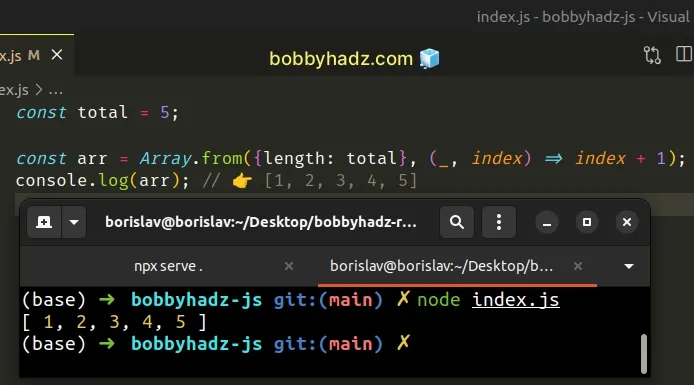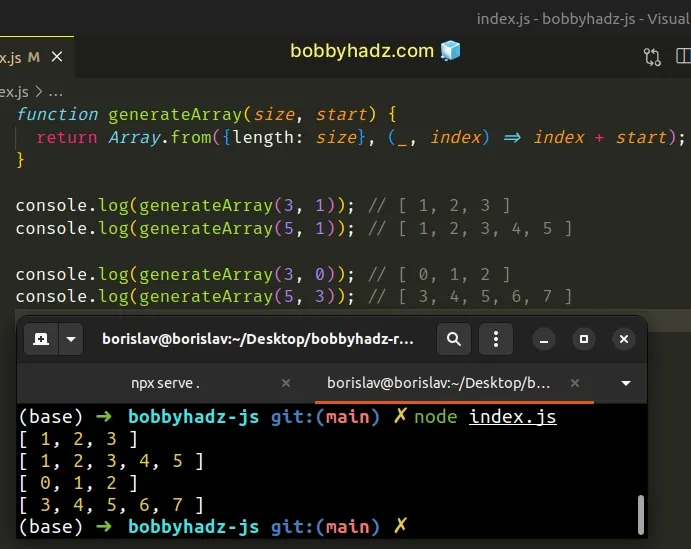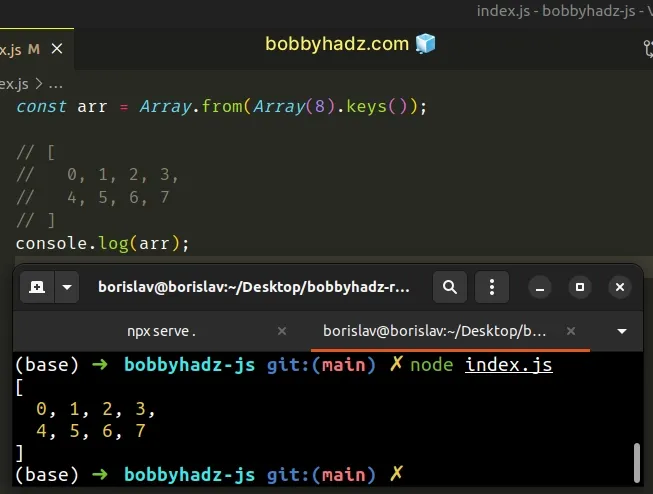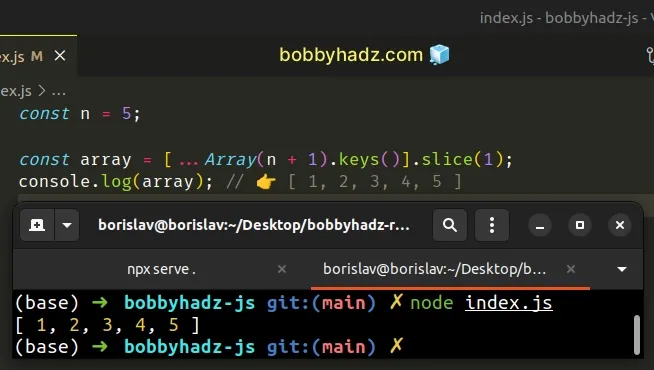# Create an Array containing 1 to N numbers in JavaScriptLast updated: Dec 21, 2022
4 min## #Create an Array containing 1 to N numbers using Array.from()

To create an array containing numbers from 1 to N:

1. Pass the desired length and a function to the `Array.from()` method.
2. The `Array.from()` method will return a new array containing the specified numbers.
index.js
```Copied!```const total = 5;

const arr = Array.from(
{length: total},
(_, index) => index + 1
);
console.log(arr) // 👉️ [1, 2, 3, 4, 5]
``````The parameters we passed to the `Array.from()` method are:

1. How many elements the array should contain.
2. A function that gets called for each element in the array.
The use of an underscore `_` character is to denote that we don't need access to the first parameter of the function, which is the value of the array element.

A call to `Array.from(3)` returns an array with 3 elements with the value of `undefined`.

We used the index of the array element in the function. Javascript indexes are zero-based, so we had to add `1` to get the desired result.

If you have to do this often, define a reusable function.

index.js
```Copied!```function generateArray(size, start) {
return Array.from({length: size}, (_, index) => index + start);
}

console.log(generateArray(3, 1)); // [ 1, 2, 3 ]
console.log(generateArray(5, 1)); // [ 1, 2, 3, 4, 5 ]

console.log(generateArray(3, 0)); // [ 0, 1, 2 ]
console.log(generateArray(5, 3)); // [ 3, 4, 5, 6, 7 ]
``````The function takes the desired size of the array and the start value as arguments and generates an array of values from `start` to N.

## #Creating an array from 0 to N with the use of keys()

If you want to create an array from `0` to N, you can also use the `Array()` constructor.

index.js
```Copied!```const arr = Array.from(Array(8).keys());

// [
//   0, 1, 2, 3,
//   4, 5, 6, 7
// ]
console.log(arr);
``````We used the `Array()` constructor to create an array of 8 empty elements.

The next step is to use the `Array.keys()` method to get an iterable of the keys (indexes) in the array.

index.js
```Copied!```// [ <8 empty items> ]
console.log(Array(8));

const arr = Array.from(Array(8).keys());

// [
//   0, 1, 2, 3,
//   4, 5, 6, 7
// ]
console.log(arr);
``````

The last step is to use the `Array.from()` method to convert the iterable to an array.

## #Create an Array containing 1 to N numbers using the spread syntax (...)

An alternative to using the `Array.from()` method is to use the spread syntax (...).

index.js
```Copied!```const n = 5;

const array = [...Array(n + 1).keys()].slice(1);
console.log(array); // 👉️ [ 1, 2, 3, 4, 5 ]
``````We used the spread syntax (...) to unpack the elements of the iterable into a new array.

We used the `Array.keys()` method, so the elements start at `0`.

To get an array starting at `1`, we had to use `slice()` to omit the first element from the result.

Alternatively, you can chain a call to the `Array.map()` method.

index.js
```Copied!```const arr = [...Array(8).keys()].map(index => index + 1);

// [
//   1, 2, 3, 4,
//   5, 6, 7, 8
// ]
console.log(arr);
``````

The function we passed to the `Array.map()` method gets called with each element of the array.

On each iteration, we increment the index by `1` and return the result.

If you want to start the array at `0`, use the `Array.keys()` method directly.

index.js
```Copied!```const arr = [...Array(8).keys()];

// [
// 0, 1, 2, 3,
// 4, 5, 6, 7
// ]
console.log(arr);
``````

## #Create an Array containing 1 to N numbers using a `for` loop

This is a three-step process:

1. Create an empty array that will hold the numbers.
2. Use a `for` loop to iterate N times, starting at `1`.
3. On each iteration, push the current index into the array.
index.js
```Copied!```const arr = [];

const total = 5;

for (let i = 1; i <= total; i++) {
arr.push(i);
}

console.log(arr); // 👉️ [1, 2, 3, 4, 5]
``````

We used a `for` loop to iterate N times, pushing the value of the `i` variable into the array.

## #Create an Array containing 1 to N numbers using `lodash`

If you use the `lodash` library, you can also use the lodash.range method to create an array from 1 to N.

index.js
```Copied!```import _ from 'lodash';

console.log(_.range(5)); // 👉️ [ 0, 1, 2, 3, 4 ]

console.log(_.range(1, 5)); // 👉️ [ 1, 2, 3, 4 ]

console.log(_.range(1, 6, 2)); // 👉️ [ 1, 3, 5 ]
``````

If you need to install `lodash`, run the following command.

shell
```Copied!```# 👇️ initialize a package.json file if you don't have one
npm init -y

npm install lodash
``````

The `lodash.range` method takes 3 arguments:

1. The start of the range. Defaults to `0`.
2. The end of the range.
3. An optional step value to increment by.